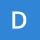Home Communities
IT Knowledge
Inspiration
Languages
EN

# JavaScript - canvas animations

0 points
Created by:DEX7RA
280

In this article, we would like to show you how to make canvas animations using JavaScript.

## 1. Basic concept

Once the shape is drawn it stays that way. In order to move it, we need to redraw the shape and everything that was drawn before,

To draw a frame you need to:

1. clear the canvas,
2. save the canvas state,
3. draw animated shapes,
4. restore canvas state.

## 2. Practical example using `requestAnimationFrame()`

In this example, we create an animation using `requestAnimationFrame()` method.

With these approaches, the frames are being drawn at the most appropriate moment for the browser.

### Simple example

This example doesn't contain any logic controlling the speed of the animation.

``````// ONLINE-RUNNER:browser;

<!doctype html>
<html>
<style>

#my-canvas { border: 1px solid gray; }

</style>
<body>
<canvas id="my-canvas" width="300" height="100"></canvas>
<script>

var canvas = document.querySelector('#my-canvas');
var context = canvas.getContext('2d');

var squareX = 0;
var squareY = 35;
var squareSize = 30;

function drawFrame(timestamp) {
// Hint: in practice it is good to use timestamp to control animation speed
context.clearRect(0, 0, canvas.width, canvas.height);
context.fillStyle = 'red';
context.fillRect(squareX, squareY, squareSize, squareSize);
squareX += 2; // 2px per frame drawing
if (squareX <= canvas.width - squareSize) {
requestAnimationFrame(drawFrame);
}
}

window.requestAnimationFrame(drawFrame);

</script>
</body>
</html>``````

### Complex example

Using `lastTimestamp` and `currentTimestamp` we are able to calculate how much time has passed since the last frame was drawn, so we can draw a smooth animation.

``````// ONLINE-RUNNER:browser;

<!doctype html>
<html>
<style>

#my-canvas { border: 1px solid gray; }

</style>
<body>
<canvas id="my-canvas" width="300" height="100"></canvas>
<script>

var lastTimestamp = null;

function requestFrame(currentTimestamp) {
if (lastTimestamp === null) {
lastTimestamp = currentTimestamp;
} else {
var result = drawFrame(currentTimestamp - lastTimestamp);
if (result === false) {
return;
}
lastTimestamp = currentTimestamp;
}
window.requestAnimationFrame(requestFrame);
}

window.requestAnimationFrame(requestFrame);

// Usage example:

var canvas = document.querySelector('#my-canvas');
var context = canvas.getContext('2d');

var squareX = 0;
var squareY = 35;
var squareSize = 30;

function drawFrame(dt) { // dt returns elapsed time in milleseconds since last drawing
context.clearRect(0, 0, canvas.width, canvas.height);
context.fillStyle = 'red';
context.fillRect(squareX, squareY, squareSize, squareSize);
squareX += 0.1 * dt;
if (squareX > canvas.width - squareSize) {
return false;
}
}

</script>
</body>
</html>``````

## 3. Inefficient solution using `setInterval()`

In this example, we present an alternative solution that clears the `canvas` and draws the square with a changed position (moved by `1`px) every millisecond.

``````// ONLINE-RUNNER:browser;

<!doctype html>
<html>
<style>

#my-canvas { border: 1px solid gray; }

</style>
<body>
<canvas id="my-canvas" width="300" height="100"></canvas>
<script>

var canvas = document.querySelector('#my-canvas');
var context = canvas.getContext('2d');

var squareX = 0;
var squareY = 35;
var squareSize = 30;

var interval = setInterval(function() {
context.clearRect(0, 0, canvas.width, canvas.height);
context.fillStyle = 'red';
context.fillRect(squareX, squareY, squareSize, squareSize);
squareX += 1;
if (squareX >= canvas.width - squareSize) {
clearInterval(interval);
}
}, 10);

</script>
</body>
</html>``````

## References

Join to our subscribers to be up to date with content, news and offers.